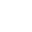# Algebra - Fun with Calendars

 June 13

 S M T W TH F S 1 2 3 4 5 6 7 8 9 10 11 12 13 14 15 16 17 18 19 20 21 22 23 24 25 26 27 28 29 30

A fun mathematical puzzle to play with your friends.
(Or

 18 19 25 26

Take any calendar. Tell your friend to choose 4 days that form a square like the four to the right. Your friend should tell you only the sum of the four days, and you can tell her what the four days are.

How does the puzzle work? You know how people always want to see a use for algebra? Well this puzzle uses algebra. Here's what I mean.

Let's pretend that the 4 numbers that the person chose were the highlighted ones here - 18, 19, 25, and 26. She adds up the four numbers and tells you only that the sum is 88.

You make a couple of calculations and tell her the numbers. What calculations? Lets figure that out with algebra. Let's call the first number n. Then you know that the next number would be n + 1 and the next number would be n + 7 and the next number would be n + 8. We had our friend add up the four numbers, so let's add our four numbers:

n + n + 1 + n + 7 + n + 8

And since our friend got 88 when she added them, let's make our sum equal 88:

n + n + 1 + n + 7 + n + 8 = 88

Simplify our equation by adding like terms:

4n + 16 = 88

How would you solve this equation? Subtract 16 from both sides?

4n = 72

Divide both sides by 4?

n = 18

Subtract 16 and divide by 4. That's exactly how you solve the puzzle. When your friend tells you the sum, you subtract 16 then divide by 4. This gives you the first number n. (Then add 1 and 7 and 8 for the other numbers).

Alternate and easier method: Subtracting 16 mentally isn't so easy. Go back to that equation:

4n + 16 = 88

I think I see a better way. Factor 4 from the left side of the equation:

4(n + 4) = 88

Now, I could divide both sides by 4:

(n + 4) = 22

Subtract 4 from both sides.

n = 18

That's a lot easier to do mentally. Divide by four and then subtract 4.

Summary: So how does the puzzle work again? Your friend adds any 4 numbers that form a square on the calendar and tells you the sum. You divide by four and then subtract 4. That gives you the first number. You add 1, 7, and 8 to get the other numbers.

And algebra makes it all possible.

Other Math Lessons by Cynthia Lanius

lanius@math.rice.edu

 StudyWebURL http://math.rice.edu/~lanius/Lessons/calen.html